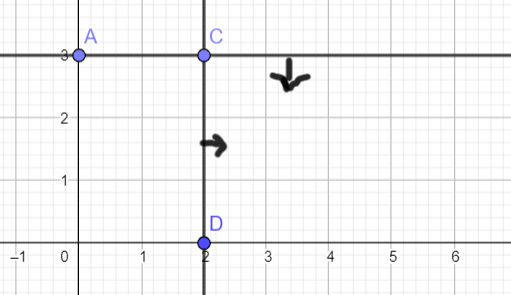# Constrained polygon ¶

Go back

It's most likely the easiest method, but it won't work if you got more than 2 parameters. Rewrite all of your constraints to have constraints like these

$\begin{split} x \le 10\\ y \le 15\\ y \le 3/5 * x + 7\\ \end{split}$

You may use greater, greater equals, or lesser/lesser equals, but you can't have something like x + y < 5 since we want only one variable on the left side.

## constrained space ¶

On you have all of your inequalities, simply trace the lines corresponding to each one. Remember to add a small arrow to visualize the constrained space.

If you have a constraint y < 3 and x > 2 it would look like thisYou should remember that something like that y <= 3/5 * x + 7 means that

• you got a point at x=0, y=7
• if x increase by 5, then y increase by 3
• so you can guess the next point (x=5, y=10)

## Minimum / Maximum ¶

The critical points are the edges of our constrained space like C in the screenshot.

You had a function f(x,y)=..., and since each point is got (x,y), then replace each critical point in the function. Take the highest (maximum) or the lowest (minimum) value, and you're done.

## Translation ¶

If you don't want to test all the points, trace your function then translate it, the last point touching it is the point you are looking for.